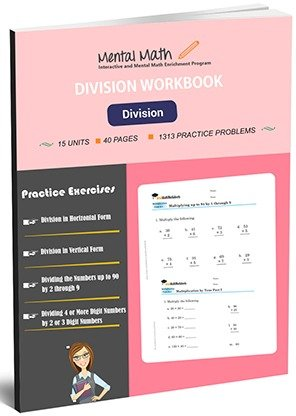20,864

# Division Worksheets\$7.00

In our math division worksheets students are introduced to the concept of division relating with the previous knowledge of multiplication. Students will learn division using numbers 2 to 9; these problems do not contain remainders in horizontal form. As they practiced with multiplication, students will divide the numbers up to 90 by 2 through 9 with or without remainders. Then, students will introduce dividing up to 4 or more digit numbers by 2 or 3 digit numbers in vertical form.

Worksheet # Topics/Curriculum # of Pages Sample Add to cart
Unit-1 Division without Remainders in Horizontal Form 8 pages\$1.00

Unit-2 Division with or without Remainders in Horizontal Form 8 pages\$1.00

Unit-3 Dividing the Numbers up to 50 by 2 through 9 8 pages\$1.00

Unit-4 Dividing the Numbers up to 60 by 2 through 9 8 pages\$1.00

Unit-5 Dividing the Numbers up to 90 by 2 through 9 8 pages\$1.00

Unit-6 Division without Remainders in Vertical Form 8 pages\$1.00

Unit-7 Dividing the Numbers up to 99 by 2 through 9 in Vertical Form 8 pages\$1.00

Unit-8 Dividing 3 Digit Numbers by 1 Digit Numbers up to 500 8 pages\$1.00

Unit-9 Dividing 3 Digit Numbers by 1 Digit Numbers up to 999 8 pages\$1.00

Unit-10 Dividing 2 or 3 Digit Numbers by 2-Digit Numbers 8 pages\$1.00

Unit-11 Dividing 2 or 3 Digit Numbers Ending at 0 or 5 8 pages\$1.00

Unit-12 Dividing 3-Digit Numbers by 2-Digit Numbers Part I 8 pages\$1.00

Unit-13 Dividing 3-Digit Numbers by 2-Digit Numbers Part II 8 pages\$1.00

Unit-14 Dividing 3 or 4 Digit Numbers by 2-Digit Numbers 8 pages\$1.00

Unit-15 Dividing 4 or More Digit Numbers by 2 or 3 Digit Numbers 8 pages\$1.00

## Choose From Over 15 Worksheets That Best Suit Your Child’s Skill Level and Unique Needs

You can purchase our high quality math worksheets separately, or purchase an entire book and save 40%!

### Equation Worksheets

Evaluate Algebraic Expressions and Solve Equations

See all units

### Integers Worksheets

Four Operations on integers and their Simplification

See all units

### Decimals Worksheets

Decimal Concept and Four Operations on Decimals

See all units

### Fractions Worksheets

Fraction Concepts and Four Operations on Fractions

See all units

### Division Worksheets

Dividing up to 4 or more digit Numbers by 2 or 3-digit Numbers

See all units

### Multiplication Worksheets

Multiplication Table of 2 through 10 and Multiplication by Thousands

See all units

### Subtraction Worksheets

Subtracting 4 to 1-digit Numbers form 4 digit Numbers

See all units